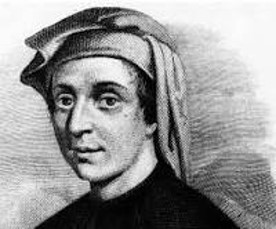## FibonacciLeonardo Fibonacci was an Italian mathematician from the Republic of Pisa, considered to be “the most talented Western mathematician of the Middle Ages”.

The name he is commonly called, Fibonacci, was made up in 1838 by the Franco-Italian historian Guillaume Libriand is short for filius Bonacci (‘son of Bonacci’). However, even earlier in 1506 a notary of the Roman Empire Perizolo mentions Leonardo as “Lionardo Fibonacci”.

Fibonacci’s 1202 manuscript Liber Abaci popularized the Hindu–Arabic numeral system in the Western World, and also introduced the sequence of Fibonacci numbers.

Fibonacci numbers

The Fibonacci numbers, commonly denoted Fₙ, are defined such that each number is the sum of the two preceding ones, starting from 0 and 1. That is, starting withthe other Fibonacci numbers are given byThe first few Fibonacci numbers are  0, 1, 1, 2, 3, 5, 8, 13, 21, 34, 55, 89, 144 ……

Here are some nice relationships between the Fibonacci numbers:Can you prove them? See if you can discover other relationships.